Publicité

# CG_U2_M2.pptx

24 Mar 2023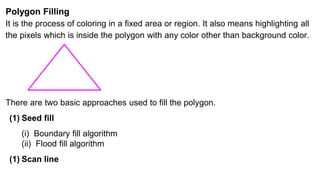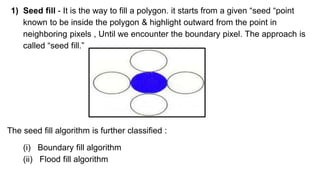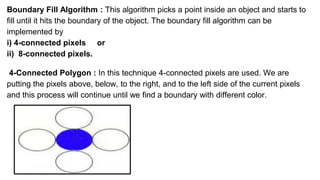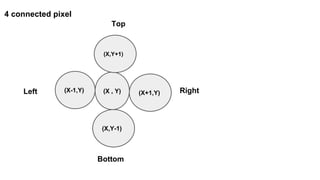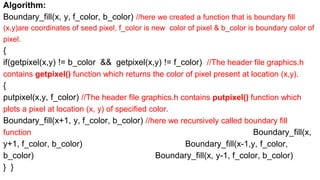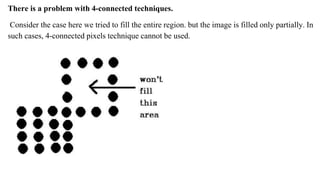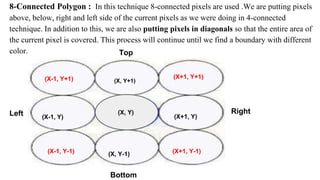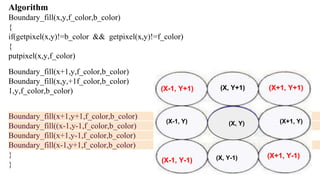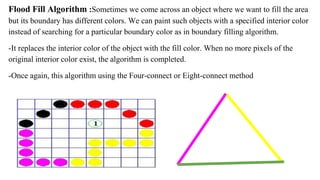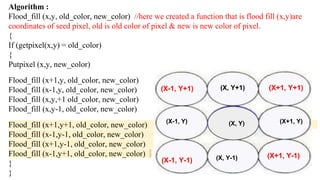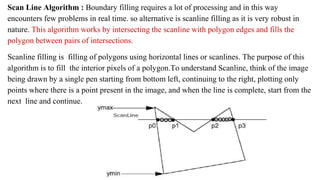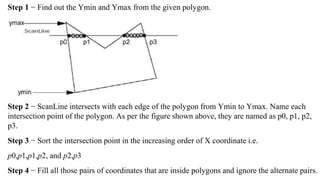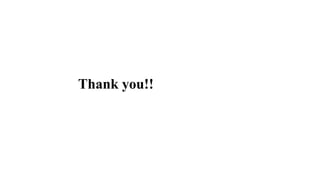1 sur 13
Publicité

### CG_U2_M2.pptx

1. Polygon Filling It is the process of coloring in a fixed area or region. It also means highlighting all the pixels which is inside the polygon with any color other than background color. There are two basic approaches used to fill the polygon. (1) Seed fill (i) Boundary fill algorithm (ii) Flood fill algorithm (1) Scan line
2. 1) Seed fill - It is the way to fill a polygon. it starts from a given “seed “point known to be inside the polygon & highlight outward from the point in neighboring pixels , Until we encounter the boundary pixel. The approach is called “seed fill.” The seed fill algorithm is further classified : (i) Boundary fill algorithm (ii) Flood fill algorithm
3. Boundary Fill Algorithm : This algorithm picks a point inside an object and starts to fill until it hits the boundary of the object. The boundary fill algorithm can be implemented by i) 4-connected pixels or ii) 8-connected pixels. 4-Connected Polygon : In this technique 4-connected pixels are used. We are putting the pixels above, below, to the right, and to the left side of the current pixels and this process will continue until we find a boundary with different color.
4. 4 connected pixel (X , Y) (X-1,Y) (X+1,Y) (X,Y-1) (X,Y+1) Right Bottom Top Left
5. Algorithm: Boundary_fill(x, y, f_color, b_color) //here we created a function that is boundary fill (x,y)are coordinates of seed pixel, f_color is new color of pixel & b_color is boundary color of pixel. { if(getpixel(x,y) != b_color && getpixel(x,y) != f_color) //The header file graphics.h contains getpixel() function which returns the color of pixel present at location (x,y). { putpixel(x,y, f_color) //The header file graphics.h contains putpixel() function which plots a pixel at location (x, y) of specified color. Boundary_fill(x+1, y, f_color, b_color) //here we recursively called boundary fill function Boundary_fill(x, y+1, f_color, b_color) Boundary_fill(x-1,y, f_color, b_color) Boundary_fill(x, y-1, f_color, b_color) } }
6. There is a problem with 4-connected techniques. Consider the case here we tried to fill the entire region. but the image is filled only partially. In such cases, 4-connected pixels technique cannot be used.
7. 8-Connected Polygon : In this technique 8-connected pixels are used .We are putting pixels above, below, right and left side of the current pixels as we were doing in 4-connected technique. In addition to this, we are also putting pixels in diagonals so that the entire area of the current pixel is covered. This process will continue until we find a boundary with different color. (X, Y+1) (X+1, Y) (X, Y-1) (X-1, Y) (X, Y) Top Bottom Left Right (X-1, Y+1) (X-1, Y-1) (X+1, Y-1) (X+1, Y+1)
8. Algorithm Boundary_fill(x,y,f_color,b_color) { if(getpixel(x,y)!=b_color && getpixel(x,y)!=f_color) { putpixel(x,y,f_color) Boundary_fill(x+1,y,f_color,b_color) Boundary_fill(x,y,+1f_color,b_color) Boundary_fill(x- 1,y,f_color,b_color) Boundary_fill(x,y-1,f_color,b_color) Boundary_fill(x+1,y+1,f_color,b_color) Boundary_fill((x-1,y-1,f_color,b_color) Boundary_fill(x+1,y-1,f_color,b_color) Boundary_fill(x-1,y+1,f_color,b_color) } } (X, Y) (X-1, Y+1) (X, Y+1) (X+1, Y+1) (X-1, Y) (X+1, Y) (X-1, Y-1) (X, Y-1) (X+1, Y-1)
9. Flood Fill Algorithm :Sometimes we come across an object where we want to fill the area but its boundary has different colors. We can paint such objects with a specified interior color instead of searching for a particular boundary color as in boundary filling algorithm. -It replaces the interior color of the object with the fill color. When no more pixels of the original interior color exist, the algorithm is completed. -Once again, this algorithm using the Four-connect or Eight-connect method
10. Algorithm : Flood_fill (x,y, old_color, new_color) //here we created a function that is flood fill (x,y)are coordinates of seed pixel, old is old color of pixel & new is new color of pixel. { If (getpixel(x,y) = old_color) { Putpixel (x,y, new_color) Flood_fill (x+1,y, old_color, new_color) Flood_fill (x-1,y, old_color, new_color) Flood_fill (x,y,+1 old_color, new_color) Flood_fill (x,y-1, old_color, new_color) Flood_fill (x+1,y+1, old_color, new_color) Flood_fill (x-1,y-1, old_color, new_color) Flood_fill (x+1,y-1, old_color, new_color) Flood_fill (x-1,y+1, old_color, new_color) } } (X, Y) (X-1, Y+1) (X, Y+1) (X+1, Y+1) (X-1, Y) (X+1, Y) (X-1, Y-1) (X, Y-1) (X+1, Y-1)
11. Scan Line Algorithm : Boundary filling requires a lot of processing and in this way encounters few problems in real time. so alternative is scanline filling as it is very robust in nature. This algorithm works by intersecting the scanline with polygon edges and fills the polygon between pairs of intersections. Scanline filling is filling of polygons using horizontal lines or scanlines. The purpose of this algorithm is to fill the interior pixels of a polygon.To understand Scanline, think of the image being drawn by a single pen starting from bottom left, continuing to the right, plotting only points where there is a point present in the image, and when the line is complete, start from the next line and continue.
12. Step 1 − Find out the Ymin and Ymax from the given polygon. Step 2 − ScanLine intersects with each edge of the polygon from Ymin to Ymax. Name each intersection point of the polygon. As per the figure shown above, they are named as p0, p1, p2, p3. Step 3 − Sort the intersection point in the increasing order of X coordinate i.e. p0,p1,p1,p2, and p2,p3 Step 4 − Fill all those pairs of coordinates that are inside polygons and ignore the alternate pairs.
13. Thank you!!
Publicité